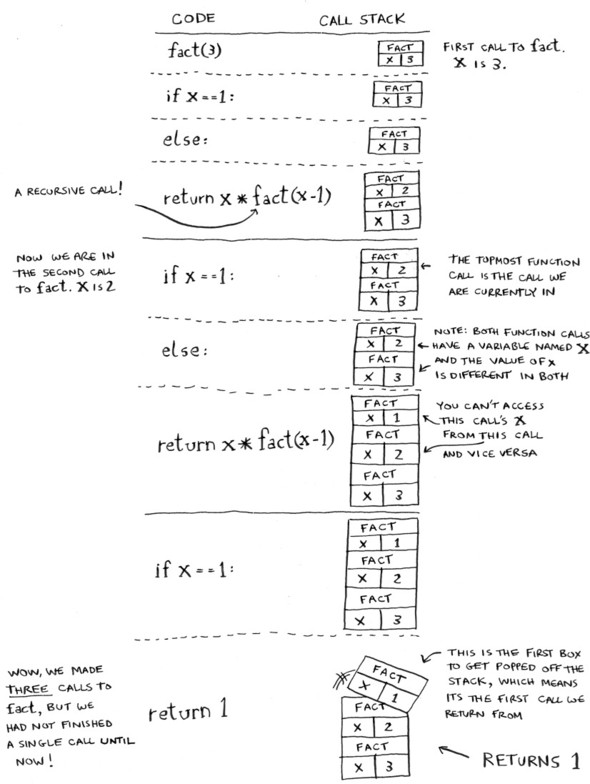To understand recursion, you must first understand recursion.

• should be used when it makes the solution clearer
• there is no performance benefit to using recursion; in fact loops are sometimes better for performance

Every recursive function should have two parts:

• base case - the function doesn’t call itself
• recursive case - the function calls itself

Wrong (no base case -> infinite loop):

``````def countdown(i):
print(i)
countdown(i-1)
``````

Right:

``````def countdown(i):
print(i)
if i <= 0:    # base case
return
else:         # recursive case
countdown(i-1)
``````

The call stack with recursions (from “Grokking Algorithms”)

``````def fact(x):
if x == 1:
return 1
else:
return x * fact(x-1)
``````